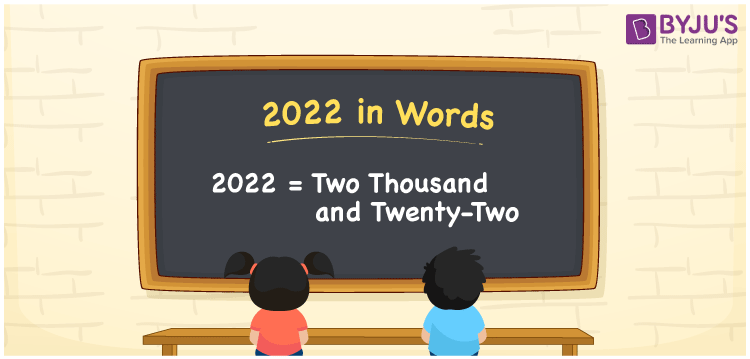# 2022 in Words

2022 can be expressed in words as Two Thousand and Twenty-Two. For instance, Sonal purchased a book worth Rs. 2022, then you can say, “Sonal purchased a book worth Rupees Two Thousand and Twenty-Two”. 2022 is a cardinal number. Students are preferred to use a place value table to convert the numbers to words easily. Hence, a place value chart is essential to represent the number names. Learn more information about how to convert the number 2022 to words and also how to spell in English in this article.

 2022 in Words Two Thousand and Twenty-Two Two Thousand and Twenty-Two in numerical form 2022

## 2022 in English Words

Generally, we represent the numbers in words using the letters of the English alphabet. Hence, we can read the number 2022 in English words as Two Thousand and Twenty-Two.## How to Write 2022 in Words?

To convert the number to words with ease, we use a place value chart. Check the place value chart for the number 2022 and its expanded form is given below.

 Thousands Hundreds Tens Ones 2 0 2 2

Therefore, we can write the expanded form as:

2 x Thousand + 0 x Hundred + 2 x Ten + 2 x One

= 2 x 1000 + 0 x 100 + 2 x 10 + 2 x 1

= 2000 + 0 + 20 + 2

= 2000 + 20 + 2

= 2022

= Two Thousand and Twenty-Two

Therefore, 2022 in words is written as Two Thousand and Twenty-Two

Interesting way of writing 2022 in words

2 = Two

20 = Twenty

202 = Two Hundred and Two

2022 = Two Thousand and Twenty-Two

Thus, the word form of the number 2022 is Two Thousand and Twenty-Two

2022 is a natural number that is the successor of 2021 and the predecessor of 2023

• 2022 in words – Two Thousand and Twenty-Two
• Is 2022 an odd number? – No
• Is 2022 an even number? – Yes
• Is 2022 a perfect square number? – No
• Is 2022 a perfect cube number? – No
• Is 2022 a prime number? – No
• Is 2022 a composite number? – Yes

## Frequently Asked Questions on 2022 in Words

Q1

### How do you write 2022 in words?

2022 in words is written as Two Thousand and Twenty-Two.
Q2

### What is the value of 3000 – 978 in words?

3000 – 978 = 2022. Hence, the value of 3000 – 978 in words is Two Thousand and Twenty-Two.
Q3

### 2022 is an even number. True or False.

True, the number 2022 is an even number.